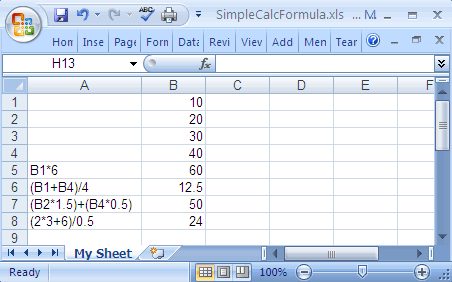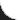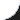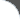Home | Tutorials | Articles | Videos | Products | Tools | Search
 Interviews | Open Source | Tag Cloud | Follow Us | Bookmark | Contact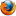In Browser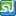StumbleUpon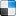del.icio.usGoogle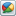Google Buzz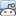redditLinkedInFacebook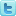TwitterLinkedinE-Mail
 Excel > JExcel API > How to perform Calucations in Excel Spreadsheet

# How to perform Calucations in Excel Spreadsheet

Java Excel API is an open source java library to read, write and modify Excel spread sheets. This requires the library jxl-2.6.12.jar to be in classpath. The following example shows how to perform simple calculations in Excel Spreadsheet.

 File Name  : com/bethecoder/tutorials/jexcelapi/write/SimpleCalcFormulaTest.java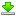Author  : Sudhakar KV Email  : [email protected]

 ``` package com.bethecoder.tutorials.jexcelapi.write; import java.io.File; import java.io.IOException; import jxl.Workbook; import jxl.read.biff.BiffException; import jxl.write.Formula; import jxl.write.Label; import jxl.write.Number; import jxl.write.WritableSheet; import jxl.write.WritableWorkbook; import jxl.write.WriteException; import jxl.write.biff.RowsExceededException; public class SimpleCalcFormulaTest {   /**    * @param args    * @throws IOException     * @throws IOException     * @throws WriteException     * @throws BiffException     */   public static void main(String[] args) throws IOException, WriteException {     //Creates a writable workbook with the given file name     WritableWorkbook workbook = Workbook.createWorkbook(new File("C:/JXL/SimpleCalcFormula.xls"));     WritableSheet sheet = workbook.createSheet("My Sheet", 0);     Number numCell = new Number(1, 0, 10);     sheet.addCell(numCell);     Number numCell2 = new Number(1, 1, 20);     sheet.addCell(numCell2);          Number numCell3 = new Number(1, 2, 30);     sheet.addCell(numCell3);          Number numCell4 = new Number(1, 3, 40);     sheet.addCell(numCell4);          addFormulaCells(sheet, "B1*6", 4);     addFormulaCells(sheet, "(B1+B4)/4", 5);     addFormulaCells(sheet, "(B2*1.5)+(B4*0.5)", 6);     addFormulaCells(sheet, "(2*3+6)/0.5", 7);          //Writes out the data held in this workbook in Excel format     workbook.write();      //Close and free allocated memory      workbook.close();    }   private static void addFormulaCells(       WritableSheet sheet,        String formula, int row) throws RowsExceededException, WriteException {          //Create a formula for average     Formula formulaCell = new Formula(1, row, formula);     sheet.addCell(formulaCell);          //Create label for average     Label formulaLabel = new Label(0, row, formula);     sheet.addCell(formulaLabel);   } }```

It gives the following output,